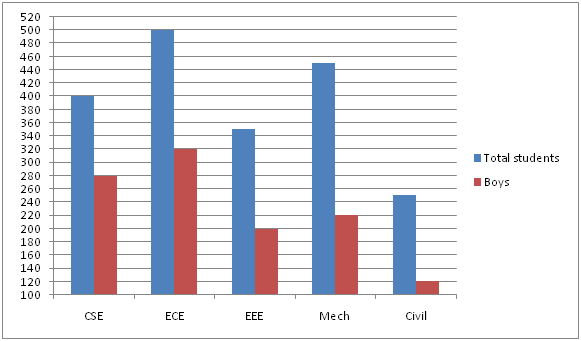# SBI Clerk Pre Quantitative Aptitude (Day-40)

Dear Aspirants, Our IBPS Guide team is providing new series of Quantitative Aptitude Questions for SBI Clerk Prelims 2020 so the aspirants can practice it on a daily basis. These questions are framed by our skilled experts after understanding your needs thoroughly. Aspirants can practice these new series questions daily to familiarize with the exact exam pattern and make your preparation effective.

Start Quiz

Ensure Your Ability Before the Exam – Take SBI Clerk 2020 Prelims Free Mock Test

Application Sums

1) A, B and C can complete 60%, 75% and 80% of the work respectively in 15 days, 12 days and 16 days respectively. In how many days A, B and C together can complete the work?

a) 4(34/61) days

b) 5(34/61) days

c) 7(34/61) days

d) 6(34/61) days

e) None of these

2) The average weight of the class is 48 kg. If two new students joined the class, then the average weight of the class increased by 1 kg and the average weight of that two new students is 56.5 kg. What is the initial number of students in the class?

a) 12

b) 15

c) 17

d) 16

e) None of these

3) The ratio of the income of A and B is 4:5 and the ratio of the expenditure of A and B is 3:2. If the total savings of A and B is Rs.2000, then what is the income of A?

a) Rs.40000

b) Rs.20000

c) Rs.16000

d) Rs.8000

e) Cannot be determine

4) If the area of the equilateral triangular area is 16√3 cm2 and the side of the triangle is 2 cm less than the breadth of the rectangle whose area is 140 cm2, then what is the perimeter of the rectangle?

a) 36 cm

b) 46 cm

c) 48 cm

d) 42 cm

e) None of these

5) If two times of the first number is subtracted from 3 times of second number is 24 and the difference between first and second number is 3. If second number is greater than first number, then find second number?

a) 13

b) 15

c) 18

d) 20

e) None of these

Data Interpretation

Directions (6 – 10): Study the following information carefully and answer the questions given below?

The given bar graph shows the total number of students and number of boys in five different departments in a college in 2018.6) The number of girls in civil department is approximately what percent of the total number of students in that department?

a) 50%

b) 51%

c) 52%

d) 53%

e) None of these

7) What is the ratio of the number of boys to girls in CSE and ECE department together?

a) 3:2

b) 2:1

c) 4:3

d) 5:4

e) None of these

8) What is the average number of girls in all the departments together?

a) 158

b) 161

c) 160

d) 162

e) 155

9) What is the difference between the number of boys and girls in EEE department?

a) 30

b) 50

c) 80

d) 100

e) 40

10) What is the difference between the average number of boys and girls in all the departments together?

a) 56

b) 58

c) 62

d) 64

e) None of these

Directions (1-5) :

A = 100/60 * 15 = 25

B = 100/75 * 12 = 16

C = 100/80 * 16 = 20

A + B + C = 1/25 + 1/16 + 1/20

= 16 + 25 + 20/400

= 61/400

Required time = 400/61

= 6(34/61) days

Total number of students = x

Total weight of the class = 48 * x

Total weight of new students = 56.5 * 2 = 113

48x + 113/(x + 2) = 49

48x + 113 = 49x + 98

x = 15

The savings of A and B is not given individually. Total savings of A and B only given. So, we can’t determine the income of A.

√3/4 * a2 = 16√3

a = 8 cm

Breadth of the rectangle = 8 + 2 = 10 cm

Length of the rectangle = 140/10 = 14 cm

Perimeter of the rectangle = 2 * (10 + 14)

= 48 cm

First number = x

Second number = y

3y – 2x = 24 ——- (1)

y – x = 3 ——-(2)

(2) * 2 – (1)

-y = -18

Y = 18

Directions (6-10) :

Required percentage = (250 – 120)/250 * 100 = 52%

Number of girls in CSE = 400 – 280 = 120

Number of girls in ECE = 500 – 320 = 180

Required ratio = (280 + 320):(120 + 180)

= 600:300

= 2:1

Girls in CSE = 400 – 280 = 120

Girls in ECE = 500 – 320 = 180

Girls in EEE = 350 – 200 = 150

Girls in Mech = 450 – 220 = 230

Girls in Civil = 250 – 120 = 130

Required Average = (120 + 180 + 150 + 230 + 130)/5

= 162

Number of girls in EEE = 350 – 200 = 150

Required difference = 200 – 150 = 50

Girls in CSE = 400 – 280 = 120

Girls in ECE = 500 – 320 = 180

Girls in EEE = 350 – 200 = 150

Girls in Mech = 450 – 220 = 230

Girls in Civil = 250 – 120 = 130

Average of girls = (120 + 180 + 150 + 230 + 130)/5

= 162

Average of boys = (280 + 320 + 200 + 220 + 120)/5 = 228

Difference = 228 – 162 = 66

 Check Here to View SBI Clerk Prelims 2020 Quantitative Aptitude Questions Day 39 Day 38 Day 37 Click Here for SBI Clerk 2020 – Detailed Exam Notification